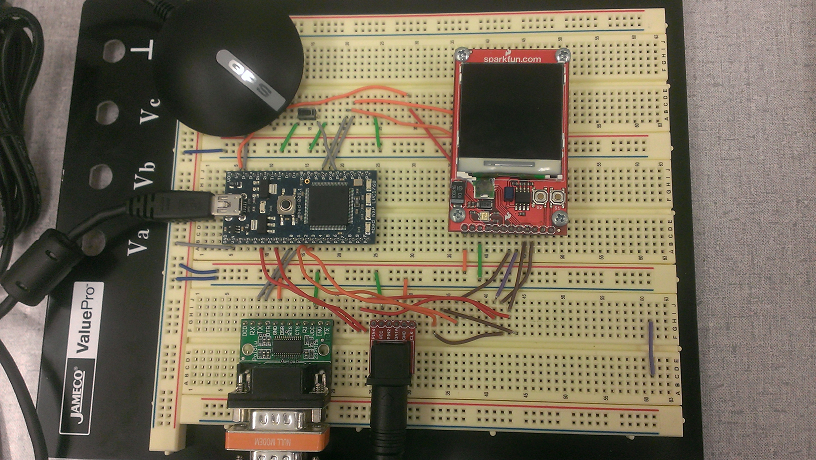## Introduction

This project has the mbed use a BR-355 GPS and a Nokia 6100 LCD to record and display distance moved. A device such as this is very practical in airplanes, automobiles and could even be used for bikers/runners.

## Description

• Once the project is started up a menu gives two options, button 1 (s1) for coordinates and button 2 (s2) for distance.
• If button 1 is pressed, the GPS coordinates are updated and displayed on the LCD every second.
• If button 2 is pressed, a second menu is displayed, giving two options: button 1 for Start/Stop and button 2 to reset recording.
• Once recording of distance starts, the updated distance is displayed on the LCD every 5 seconds.
• Button 1 can be held down while distance is being calculated to pause distance calculation.
• Button 2 can be pressed to reset the distance calculation.
• The third push-button (the one not on the LCD) can be pressed at any time to return to the first menu.
• If the GPS can not find coordinates the LCD will display "No Lock"

## Hardware

1. mbed
2. BR-355 GPS Module
3. Sparkfun Nokia 6100 Color LCD Breakout Board
4. RS-232 Breakout Board
5. PS/2 Breakout Board
6. push button

## ConnectionsNokia 6100 LCDMbed
VBATTVV = 5V
3.3VVout (3.3.V)
GndGnd
Resetp12
DIOp5
SCKp7
CSp11
S2p27
S1p28

#### Note

The LCD's on board push-buttons (S1 and S2) are used, but any standard push-button's could be used.

PS/2Mbed
VccVV=5V
GndGnd
RS-232Mbed
VccVV = 5V
GndGnd
TXp10
RXp9

#### Information

You might need to use a mini gender changer and NULL modem with Sparkfun RS-232 breakout. Also, depending on the RS-232 model, wiring RX may or may not be required, even if you aren't using it.

## Code

The following API's were used with this code:

Distance Calculation Info:

#### main.cpp

```#include "mbed.h"
#include "GPS.h"
#include "NokiaLCD.h"
#include <math.h>
#define PI 3.14159265

NokiaLCD lcd(p5, p7, p11, p12, NokiaLCD::LCD6610); // mosi, sclk, cs, rst, type
GPS gps(p9, p10);
DigitalIn pb1(p28);
DigitalIn pb2(p27);
DigitalIn pbReset(p26);

void setup();

void coordinates()
{

while(1) {
if (!pbReset) {
setup();
}
lcd.cls();
lcd.locate(0,3);
//display coordinates every 1 second
if(gps.sample()) {
lcd.printf("Location at %f, %f", gps.longitude, gps.latitude);

} else {
lcd.printf("No Lock");
}
wait(1);
}

}

void distance()
{
float dist = 0;
float lat2 = 0;
float lon2 = 0;
float lat1 = 0;
float lon1 = 0;
float dLat = 0;
float dLon = 0;
float c = 0;
float a = 0;
float d = 0;
lcd.cls();
lcd.locate(0,3);
lcd.printf("1 : Start/Stop");
lcd.locate(0,5);
lcd.printf("2 : Reset Recording");
wait(1);
//Wait for buttons to be pressed
while( pb2 && pb1 && pbReset) {
}
wait(.001);
if (!pbReset) {
setup();
}
if (!pb2) {
distance();
}
if (!pb1) {
//Sample First Value
if (gps.sample()) {
lat1 = gps.latitude;
lon1 = gps.longitude;
lcd.cls();
lcd.locate(0,3);
lcd.printf("Signal Locked");
wait(5);
// Begin Calculating Distance
while(1) {
if (!pbReset) {
setup();
}
// Reset Distance Calculation if pb2 pushed
if (!pb2) {
distance();
}
// Stop Recording while pb1 is pushed
while(!pb1) {
lcd.locate(0,3);
lcd.printf("Stopped");
wait(.3);
}

//Sample Second Value
if (gps.sample()) {
lat2 = gps.latitude;
lon2 = gps.longitude;
}

//Calculate Distance
dLat = (lat2-lat1)*(PI/180);
dLon = (lon2-lon1)*(PI/180);
a = sin(dLat/2) * sin(dLat/2) +
sin(dLon/2) * sin(dLon/2) * cos(lat1*(PI/180)) * cos(lat2*(PI/180));
c = 2 * atan2(sqrt(a), sqrt(1-a));
d = 6371000 * c;

//GPS is only precise to 5 meters, so throw out bad data
if ( d <= 5) {
d = 0;
}
dist = dist + d;
lcd.cls();
lcd.locate(0,3);
lcd.printf("Distance Moved: %f", dist);
lat1 = lat2;
lon1 = lon2;

//Sample Every 5 Seconds
wait(5);
}
} else {
lcd.cls();
lcd.locate(0,3);
lcd.printf("No lock");
wait(3);
distance();
}

}
}

void setup()
{
lcd.background(0x0000FF);
lcd.cls();
lcd.locate(0,3);
lcd.printf("1 : Coordinates");
lcd.locate(0,5);
lcd.printf("2 : Distance");
lcd.locate(0,7);
while( pb2 && pb1) {
}
wait(.001);
if (!pb1) {
coordinates();

}
if (!pb2) {
distance();
}
lcd.cls();
}

int main()
{
pb2.mode(PullUp);
pb1.mode(PullUp);
pbReset.mode(PullUp);
setup();
}
```

## Video

#### Troubleshooting!

The BR-355 may not work inside buildings, and get's best reception in open areas. Also, the GPS is only accurate to about 5 meters, therefore if you are moving slower than 1 meter per second, your movement may not be added to the displayed distance.